Courses

# Probability MCQ Quiz - 2

## 30 Questions MCQ Test RRB JE for Computer Science Engineering | Probability MCQ Quiz - 2

Description
This mock test of Probability MCQ Quiz - 2 for Computer Science Engineering (CSE) helps you for every Computer Science Engineering (CSE) entrance exam. This contains 30 Multiple Choice Questions for Computer Science Engineering (CSE) Probability MCQ Quiz - 2 (mcq) to study with solutions a complete question bank. The solved questions answers in this Probability MCQ Quiz - 2 quiz give you a good mix of easy questions and tough questions. Computer Science Engineering (CSE) students definitely take this Probability MCQ Quiz - 2 exercise for a better result in the exam. You can find other Probability MCQ Quiz - 2 extra questions, long questions & short questions for Computer Science Engineering (CSE) on EduRev as well by searching above.
*Answer can only contain numeric values
QUESTION: 1

### Four fair six-sided dice are rolled. The probability that the sum of the results being 22 is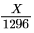. The value of X is ______

Solution:

There are only two possible sets whose elements sum to 22 : {6,6,6,4}, {6,6,5,5}
Number of permutations for 1st set : 4!/3! = 4
Number of permutations for 2nd set : 4!/(2!*2!) = 6
So total number of ways to sum 22 = 10
So X =10.

QUESTION: 2

### Let P(E) denote the probability of the event  E. Given P(A) = 1, P(B) = 1/2, the values of P(A|B) and P(B|A) and respectively are

Solution:

It immediately follows from the monotonicity property that ,
0 <= P(E) <= 1 ,
probability of atleast one means union of the probability of events , i.e.
P(A U B) = P(A) + P(B) - P(A⋂B)
here , P(AUB) = 1 , because it can not be more than 1 and if atleast one of event have probability 1 (here , P(A) = 1) , then union of both should be 1 .
so , P(A U B) = P(A) + P(B) - P(A⋂B)
1 = 1 + 1/2 - P(A⋂B) ,
P(A⋂B) = 1/2 ,
now , P(A/B) = P(A⋂B) / P(B) = (1/2) / (1/2) = 1 ,
P(B/A) = P(A⋂B) / P(A) = (1/2) / (1) = 1/2 .
hence option (D) ,
NOTE :- if atleast one of the two events has/have the probability 1 . then both events should be independent events but vise - versa not true .

QUESTION: 3

### You have to play three games with opponents A and B in a specified sequence. You win the series if you win two consecutive games. A is a stronger player than B. Which sequence maximizes your chance of winning the series?

Solution:

Let the probability of winning against player A be  and the probability of winning against player B be b.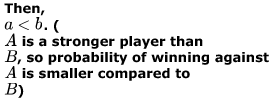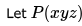be the probability of winning the series in which the games played are against x,y and z in order.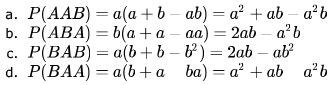We can see that not all probabilities are equal, so option E is not correct.
We can also see that options A and D result in the same value, so they are not correct either.
Comparing option B and option C.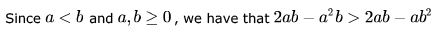Hence, option B is the correct answer.

QUESTION: 4

Three dice are rolled independently. What is the probability that the highest and the lowest value differ by 4?

Solution:

Case 1:largest is 5 , smallest 1 and middle is 2 or 3 or 4 : 3*3!
Case 2.largest is 5 , smallest 1 and middle is 1 or 5 : 3!*2/2!
Case 3:largest is 6 , smallest 2 and middle is 3 or 4 or 5 : 3*3!
Case 4:largest is 6 , smallest 2 and middle is 6 or 2: 3!*2/2!
So probability the highest and the lowest value differ by 4 =( 3*3!+3!*2/2!+3*3!+3!*2/2!)/63 =2/9

QUESTION: 5

The probability of throwing six perfect dices and getting six different faces is

Solution:

B) for all the 6 different faces 1,2,3,4,5,6 the probability is
1/66 and then for
6! different permutations :
6! / 66

QUESTION: 6

There are 1000 balls in a bag, of which 900 are black and  are white. I randomly draw 100 balls from the bag. What is the probability that the 100st ball will be black?

Solution:

Here we are having a total of 1000 Balls, out of which we firstly draw 100 balls , and then 101st ball..
Firstly we have to find expected number of white and black balls in drawn 100 balls , as both can occur in 100 balls..
We have a situation like this: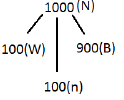Expected number of white balls = n*W/N = 100*(100/1000) = 10
Expected number of black balls = n*B/N = 100*(900/1000) = 90
So,  we have drawn 100 balls(90 black, 10 white)
Left balls = (810 Black , 90 white) = 900 total
Now,

probability for 101st ball to be black = 810/900 = 9/10
So, option (A) is Correct

QUESTION: 7

Two n bit binary strings, S1 and S2 are chosen randomly with uniform probability. The probability that the Hamming distance between these strings (the number of bit positions where the two strings differ) is equal to d is

Solution:

there n binary bits that can differ but only d should differ in this case
ways of choosing these d bits = nCd probability of d bits differ but, n - d bits do not differ = (1/2)d(1/2)(n - d)
no of ways = nCd/2n

QUESTION: 8

A biased coin is tossed repeatedly. Assume that the outcomes of different tosses are independent and probability of heads is 2/3 in each toss. What is the probability of obtaining an even number of heads in  tosses, zero being treated as an evennumber?

Solution:

probability of obtaining an even number of heads in 5 tosses, zero being treated as an even number number of event = 0 head or 2 head or 4 head
Probability of tail = 1/3
Probability =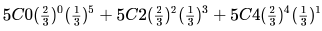= 121/243

QUESTION: 9

You are lost in the National park of Kabrastan. The park population consists of tourists and Kabrastanis. Tourists comprise two-thirds of the population the park, and give a correct answer to requests for directions with probability 3/4. The air of Kabrastan has an amnesaic quality however, and so the answers to repeated questions to tourists are independent, even if the question and the person are the same. If u ask a Kabrastani for directions, the answer is always wrong.
Suppose you ask a randomly chosen passer by whether the exit from the park is East or West. The answer is East. You then ask the same person again, and the reply is again East. What is the probability of East being correct?

Solution:

Tourists are 2/3 of population of the park
Among them probability of correct answer is 3/4
so, 2/3*3/4=1/2

QUESTION: 10

Doctors A and B perform surgery on patients in stages III and IV of a disease. Doctor A has performed a 100 surgeries (on 80 stage III and 20 stage  patients) and 80 out of her 100 patients have survived (78 stage III and 2 stage IV survivors). Doctor B has also performed 100 surgeries (on 50 stage III and 50 stage IV patients). Her success rate is  (49 stage III survivors and 11 stage IV survivors). A patient has been advised that she is equally likely to besuffering from stage III or stage IV of this disease. Which doctor would you recommend to this patient and why?

Solution:

As, %of Doctor A successful for stage III 78/80*100=97.5
for stage IV 2/20*100=10
As, %of Doctor B successful for stage III 49/50*100=98
stage IV 11/50*100=22

QUESTION: 11

An unbiased die is thrown n times. The probability that the product of numbers would be even is

Solution:

Even number = 2,4,6
odd number = 1,3,5
Product will come even when even one time even number comes odd product will be if every time odd comes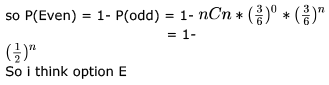QUESTION: 12

If a fair coin is tossed four times. What is the probability that two heads and two tails will result?

Solution:

out of 4 times 2 times head should be present
ways of selecting these 2 places 4C2 probability of getting 2 heads and 2 tails = (1/22)(1/22)
probability = 4C2/24 = 3/8

QUESTION: 13

Consider a -sided die with all sides not necessarily equally likely such that probability of an even number is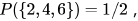, probability of a multiple of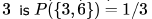and probability of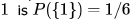. Given the above conditions, choose the strongest (most stringent) condition of the following that must always hold about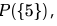, the probability of 5.

Solution:

P{3,5} = 1 - P{2,4,6} - P{1} = 1/2 - 1/6 = 1/3
Can P{3} = 0? then P{6} = 1/3 and P{2,4} = 1/2 - 1/3 = 1/6. And P{5} = 1/3. Possible. So, option D.

QUESTION: 14

Four fair coins are tossed simultaneously. The probability that at least one head and one tail turn up is

Solution:

probability of getting all heads = 1/16
probability of getting all tails = 1/16
probability of getting at least one head and one tail = 1 - 1/16 - 1/16 = 7/8

QUESTION: 15

The probability of three consecutive heads in four tosses of a fair coin is.

Solution:

Let the 4 tosses be named P,Q,R and S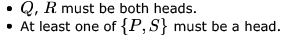Thus, the probability of getting 3 consecutive heads is given by: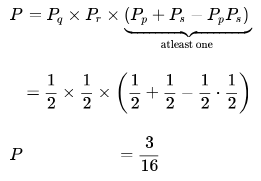Hence, option D is the correct answer.
Another way of looking at it is: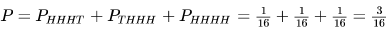*Answer can only contain numeric values
QUESTION: 16

A probability density function on the interval [a,1] is given by 1/x2 and outside this interval the value of the function is zero.
The value of a is _________.

Solution:

We know that the sum of all the probabilities is 1.
Therefore on integrating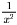with limits a to 1, the result should be 1.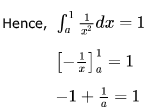Hence a = 0.5

Integrate 1/x2 in the limits a to 1.. and equate it to 1.. solving we get a=0.5

*Answer can only contain numeric values
QUESTION: 17

Consider the following experiment.
Step 1. Flip a fair coin twice.
Step 2. If the outcomes are (TAILS, HEADS) then output Y and stop.
Step 3. If the outcomes are either (HEADS, HEADS) or (HEADS, TAILS), then output N and stop.
Step 4. If the outcomes are (TAILS, TAILS), then go to Step 1.
The probability that the output of the experiment is Y is (up to two decimal places) ____________.

Solution:

1st time it is 0.25(1/4), when tail tail comes, entire process gets repeated, so next time probability of Y to happen is 0.25*0.25 ((1/4)*(1/4)), likewise it goes on as infinite GP
Sum of infinite GP = a/(1-r)
here a= 1/4 and r = 1/4
so answer becomes 1/3 i.e 0.33

P(HH + HT)=1/2
now if TT comes then toss again,
So, P(TTTH)= 1/16 . and so on.... P(TH+TTTH+........) = 1/4 + 1/16+... = 1/3

QUESTION: 18

An unbalanced dice (with 6 faces, numbered from 1 to 6) is thrown. The probability that the face value is odd is 90 of the probability that the face value is even. The probability of getting any even numbered face is the same. If the probability that the face is even given that it is greater than 3 is 0.75, which one of the following options is closest to the probability that the face value exceeds 3?

Solution: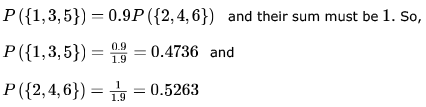Given that probability of getting 2 or  4 or 6 is same.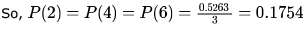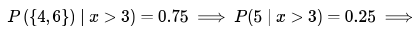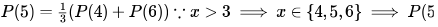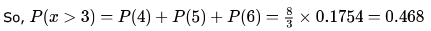QUESTION: 19

A man has three cats. At least one is male. What is the probability that all three are male?

Solution:

Given that A man has three cats and  At least one is male.
Possible combination for atleast one cat is male=(M,F,F),(F,M,F),(F,F,M),(M,F,M),(M,M,F),(F,M,M),(M,M,M)

Probability that all three are male = 1/7
Hence,Option(B)
1/7 is the correct Choice.

QUESTION: 20

The probability that a number selected at random between 100 and 999 (both inclusive) will not contain the digit 7 is:

Solution:

First digit can be chosen in 8 ways from 1−9 excluding 7
Second digit can be chosen in 9 ways from 0−9 excluding 7 and similarly the third digit in 9 ways.
So, total no. of ways excluding 7 = 8∗9∗9
Total no. of ways including 7 = 9∗10∗10
So, ans = (8∗9∗9)/(9∗10∗10)=18/25

QUESTION: 21

The 12 houses on one side of a street are numbered with even numbers starting at 2 and going up to 24. A free newspaper is delivered on Monday to 3 different houses chosen at random from these 12. Find the probability that at least 2 of these newspapers are delivered to houses with numbers strictly greater than 14.

Solution:

There are  12 houses on one side of a street are numbered with even numbers.
In which 5 houses are strictly greater than Number 14.
And remaining 7 houses are numbered smaller than 14 (i.e. including 14)
No of way of choosing at least 2 of these newspapers are delivered to houses with numbers strictly greater than 14.
5C3  +5C2 * 7C1 =80
Total way of choosing 3 houses=12C3 =220
So Required probability=80/220=4/11

QUESTION: 22

You arrive at a snack bar and you can’t decide whether to order a lime juice or a lassi. You decide to throw a fair 6-sided die to make the choice, as follows.

• If you throw 2 or 6 you order a lime juice.
• If you throw a 4, you order a lassi.
• Otherwise, you throw the die again and follow the same algorithm.

What is the probability that you end up ordering a lime juice?

Solution:

If we want lime juice then we need to throw {2 or 6} . And If we don't get {2 or 6} in first go, then we need to throw {1 or 3 or 5} in first go and again we will try to get {2 or 6} in 2nd throw and so on.
Note - If we throw 4 in any go then we will end up getting lassi. But we want lime juice.
So probability of getting lime juice = (2/6)  + (3/6) * (2/6) + (3/6)2 * (2/6) + .......  to infinity.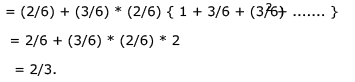QUESTION: 23

Consider the  function on three bits, maj :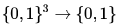where maj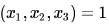if and only if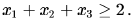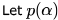be the probability that the output is 1 when each input is set to 1 independently with probability α. What is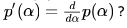Solution: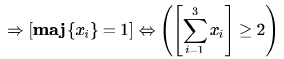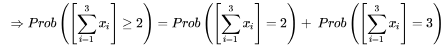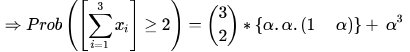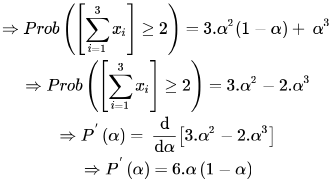QUESTION: 24

If P and Q are two random events, then the following is TRUE:

Solution:

here i check option---> i let P = probability,  P and Q is replaced by A and B.
A) it  is saying independent means P(A ^ B) =  P(A)* P(B)  so option A is false
B) P(AÜ B) =  P(A) + P(B) - P(A ^ B)
hence P(AÜB) >= P(A) + P(B)  its false option B is false
C) if A and B is mutually exclusive means P(A ^ B) =  0 then its independent ........its false  there is no relation independent and mutually exclusive
D)  P(A ^ B) <= P(A)

its true

hence option D is correct

QUESTION: 25

Seven (distinct) car accidents occurred in a week. What is the probability that they all occurred on the same day?

Solution:

for every car accident we can pick a day in 7 ways
total number of ways in which accidents can be assigned to days = 77
probability of accidents happening on a particular day = 1/77
we can choose a day in 7 ways
hence probability = 7/77= 1/76

QUESTION: 26

E1 and E2 are events in a probability space satisfying the following constraints:

• Pr(E1) = Pr(E2)
• Pr(E1 ∪ E2) = 1
• E1 and E2 are independent

The value of Pr(E1), the probability of the event E1, is

Solution:

let probability of Event E1 = x = prob of E2
prob(E1 union E2) = prob(E1) + prob(E2) - prob(E1 intersect E2)
1 = x + x -x2 (prob(E1 intersect E2) = prob(E1) * prob(E2) as events are independent)
x = 1

QUESTION: 27

The minimum number of cards to be dealt from an arbitrarily shuffled deck of 52 cards to guarantee that three cards are from same suit is

Solution:

There are 4 sets of cards. So, up till 8 cards there is a chance that no more than 2 cards are from a given set. But, once we pick the 9th one, it should make  3 cards from any one of the sets. So, (C) is the answer.

QUESTION: 28

A bag contains  white balls and  black balls. Two balls are drawn in succession. The probability that one of them is black and the other is white is:

Solution:

probability of first ball white and second one black = (10/25)x(15/24)
probability of first ball black and second one white = (15/25)x(10/24)
probabilty = sum of above two probabilities = 1/2

*Answer can only contain numeric values
QUESTION: 29

The probability that a given positive integer lying between 1 and 100 (both inclusive) is NOT divisible by 2, 3 or 5 is ______ .

Solution:

no of integers divisible by 2 = 50
no of integers divisible by 3 = 33
no of integers divisible by 5 = 20
no of integers divisible by 2 and 3 = 16
no of integers divisible by 2 and 5 = 10
no of integers divisible by 3 and 5 = 6
no of integers divisible by 2 and 3 and 5 = 3
total numbers divisible by 2 or 3 or 5 = 50 + 33 + 20 -16 -10 - 6 + 3 = 74
total number not divisible by 2 or 3 or 5 = 26
probability = 0.26 [EDIT]

QUESTION: 30

If two fair coins are flipped and at least one of the outcomes is known to be a head, what is the probability that both outcomes are heads?

Solution:

prob(at least one head) = 3/4